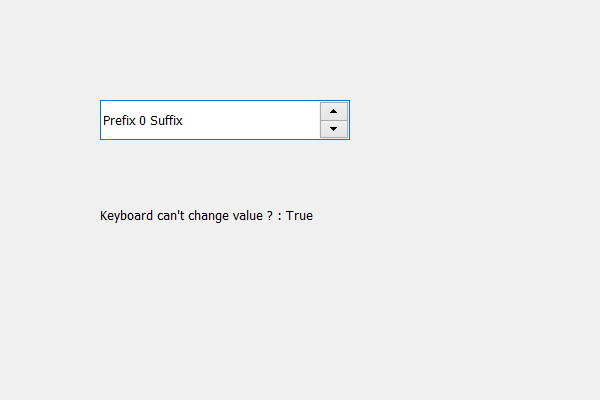# PyQt5 QSpinBox – Checking if user can change value using keyboard or not

• Last Updated : 17 May, 2020

In this article we will see how we can check if user can change the spin box value using key board or not, when we create a spin box there are basically two ways to change the value of the spin box one is by using arrow buttons or other way is using key board. We can stop keyboard input of spin box using `setReadOnly` method with its line edit object.

In order to check this we use isReadOnly method with the line edit object

Argument : It takes no argument

Return: It returns bool

In order to do this we have to do the following :
1. Create a main window
2. Create a spin box
3. Get the line edit object from the spin box
5. Create a label to show result
6. Check if line edit is read only or not
7. Show check value on screen through label

Below is the implementation

 `# importing libraries``from` `PyQt5.QtWidgets ``import` `*` `from` `PyQt5 ``import` `QtCore, QtGui``from` `PyQt5.QtGui ``import` `*` `from` `PyQt5.QtCore ``import` `*` `import` `sys`` ` ` ` `class` `Window(QMainWindow):`` ` `    ``def` `__init__(``self``):``        ``super``().__init__()`` ` `        ``# setting title``        ``self``.setWindowTitle(``"Python "``)`` ` `        ``# setting geometry``        ``self``.setGeometry(``100``, ``100``, ``600``, ``400``)`` ` `        ``# calling method``        ``self``.UiComponents()`` ` `        ``# showing all the widgets``        ``self``.show()`` ` `    ``# method for widgets``    ``def` `UiComponents(``self``):``        ``# creating spin box``        ``self``.spin ``=` `QSpinBox(``self``)`` ` `        ``# setting geometry to spin box``        ``self``.spin.setGeometry(``100``, ``100``, ``250``, ``40``)`` ` `        ``# setting prefix to spin``        ``self``.spin.setPrefix(``"Prefix "``)`` ` `        ``# setting suffix to spin``        ``self``.spin.setSuffix(``" Suffix"``)`` ` `        ``# getting the line edit``        ``line ``=` `self``.spin.lineEdit()`` ` `        ``# making the line edit part read only``        ``line.setReadOnly(``True``)`` ` `        ``# creating a label``        ``label ``=` `QLabel(``self``)`` ` `        ``# setting geometry to the label``        ``label.setGeometry(``100``, ``200``, ``300``, ``30``)`` ` `        ``# checking if line edit is read only``        ``check ``=` `line.isReadOnly()`` ` `        ``# setting text to label``        ``label.setText(``"Keyboard can't change value ? : "` `+` `str``(check))`` ` ` ` `# create pyqt5 app``App ``=` `QApplication(sys.argv)`` ` `# create the instance of our Window``window ``=` `Window()`` ` `# start the app``sys.exit(App.``exec``())`

Output :My Personal Notes arrow_drop_up